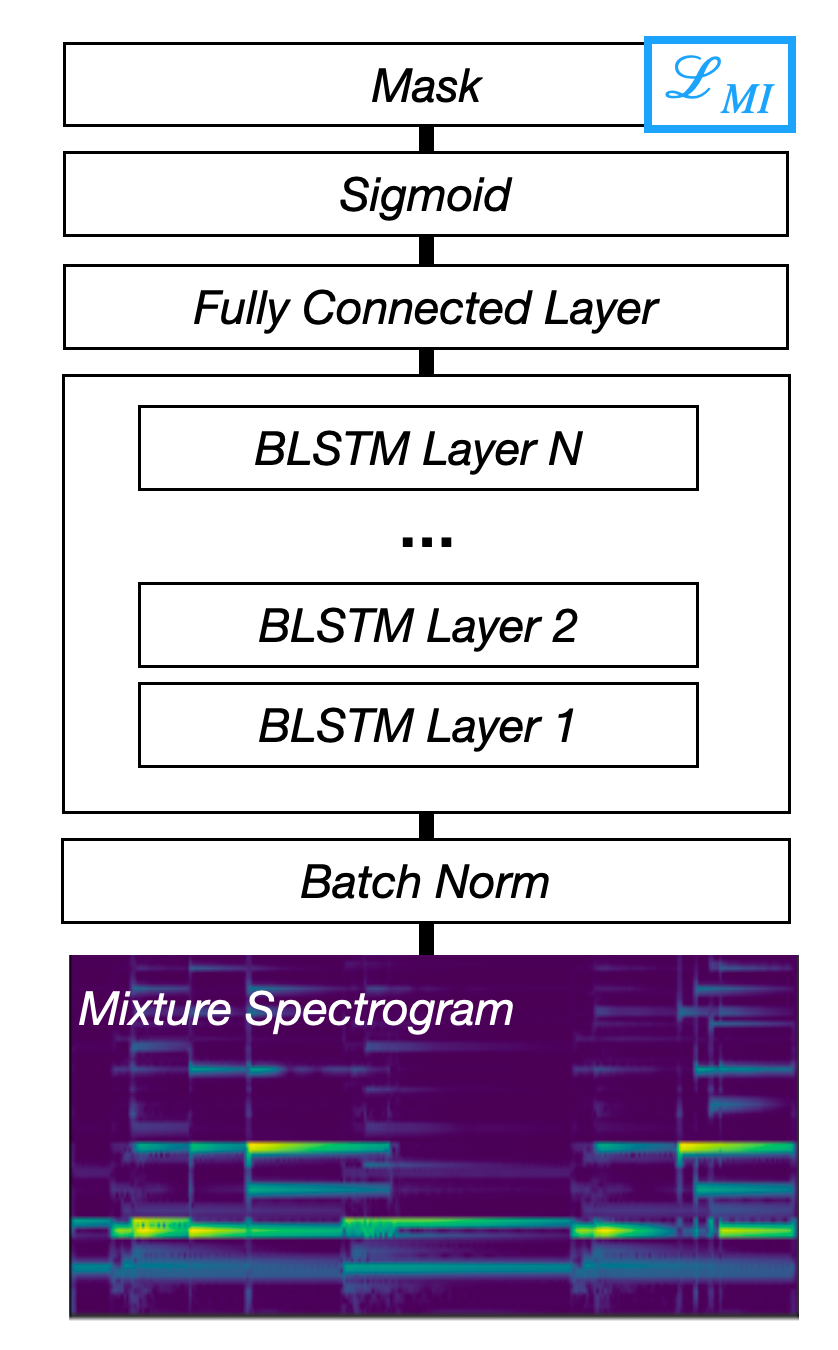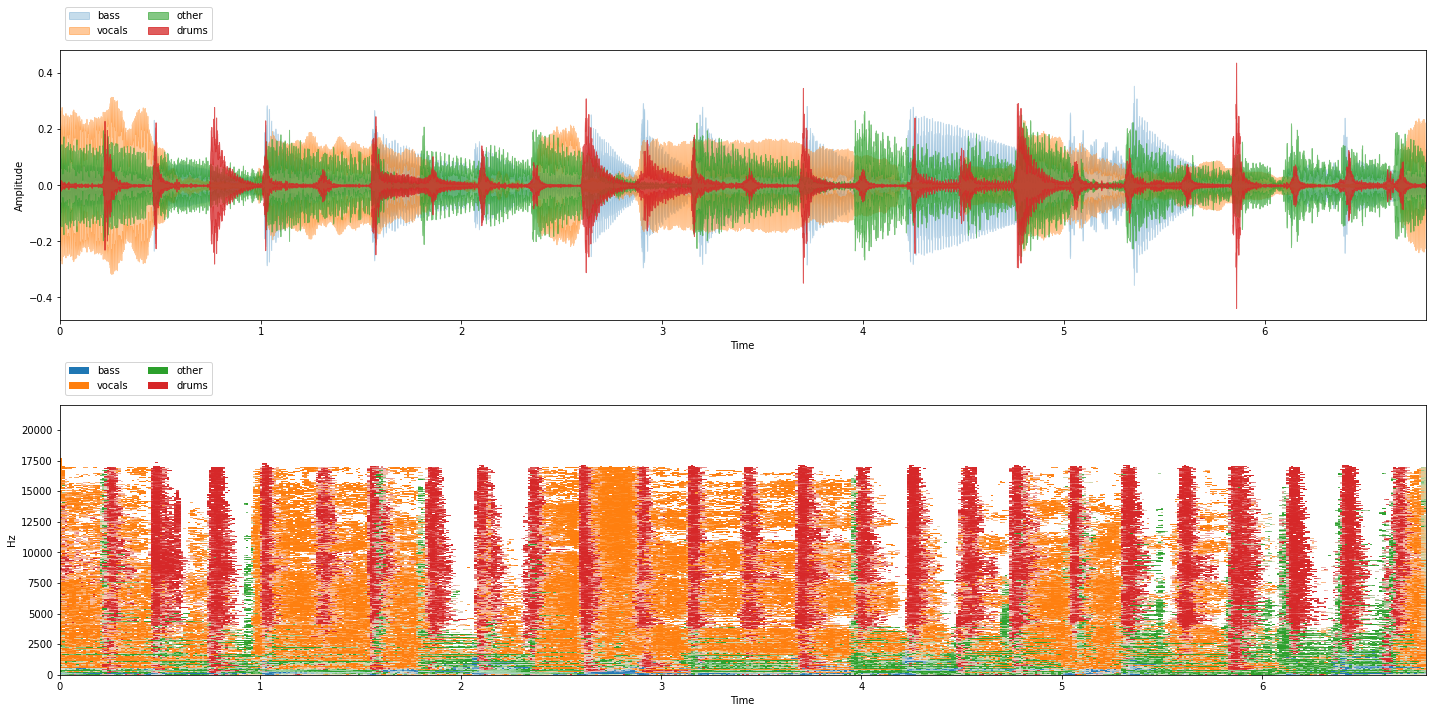# Coding up model architectures¶

Most deep separation networks that have cropped up in recent years use and re-use many of the same components. While some papers introduce new types of layers, often there are still components that can be abstracted out and used across multiple network architectures. This structure has solidified in recent years, with at least two open source projects - Asteroid and nussl - recognizing this.

This chapter is meant to acquaint you with the exact implementations of each of these building blocks, and eventually build them up to a few different, interesting, network architectures that are seen throughout the source separation literature.

%%capture
!pip install scaper
!pip install nussl
!pip install git+https://github.com/source-separation/tutorial


Our story starts with one of the more established methods for deep audio source separation - learning the mask. Recall that the goal of many audio source separation algorithms before deep learning was to construct the optimal mask that when applied to the STFT (or other invertible time-frequency representation such as the CQT) of the mixture, produced an estimate of the isolated source - vocals, accompaniment, speaker one, speaker two, etc. When deep networks first came on the scene, one obvious thing to do was to create a deep network that would predict the masks directly.Fig. 49 A diagram of the Mask Inference architecture.

Note

Some early work tried to predict the magnitudes directly, but this generally resulted in worse performance. Things may be changing though, with some recent work.

### Model overview¶

In the sections below we will be building up a deep mask estimation model. The model takes a representation of the mixture (the magnitude spectrogram), and converts it into a mask with the following steps:

1. Convert magnitude spectrogram to log-magnitude spectrogram.

2. Normalize the log-magnitude spectrogram.

3. Process the log-magnitude spectrogram with a stack of recurrent neural networks.

4. Convert the output of the recurrent stack to a mask that can be applied to the mixture.

This is a “simple” model that is for mostly pedagogical purposes, but does work well for some separation problems. We’ll look at more complicated models later on.

We’ll start with the last step, so that we understand the output of the network. Then, we’ll learn how to do the first 3 steps. A model in PyTorch always looks like this:

import torch
from torch import nn
import nussl

nussl.utils.seed(0)
to_numpy = lambda x: x.detach().numpy()
to_tensor = lambda x: torch.from_numpy(x).reshape(-1, 1).float()

class Model(nn.Module):
def __init__(self):
super().__init__()

def forward(self, data):
return data


In the __init__ function, you save everything the model needs to do its computation. This includes initialized building blocks, and any other variables needed. In the forward function, you define the actual data flow of the model as it goes from input to output (in this case a mask).

The first building block we’ll look at is one of the simplest ones - a mask. As we saw in previous chapters, one way to perform separation is to element-wise multiply a mask with a representation. This representation is generally the magnitude spectrogram. We’ll start by introducing it in numpy, and then move towards doing it in PyTorch.

import torch
import nussl
from common import viz
import numpy as np
import matplotlib.pyplot as plt